# Two Variable Data Table in Excel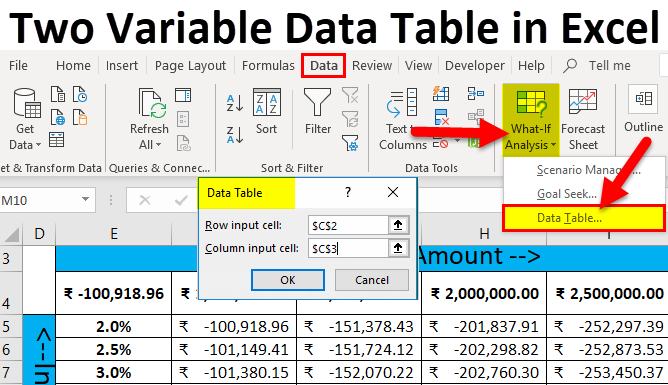• Two-Variable Data Table in Excel
• How to Perform Two-Variable Data Table in Excel?

## Two-Variable Data Table in Excel

Two variable data Table is a feature in MS Excel, which is used when a user wants to see multiple results with two Input variables. The best use of two variable data table is when there is a requirement for the change two input parameters at the same time and calculate the resultant for same.

As it is clear with the name itself there are two variables required for the data table. In simple words, we can say how two changing inputs changed the one output.

It is a part of the What-If analysis tool in MS Excel, which is the best in analyses during continues changes of the input parameter.

As an example, Loan re-payment- there is one input parameter interest rate and another one is a year. A user can see a different scenario by changing input parameters.

### How to Perform Two-Variable Data Table in Excel?

Two-Variable Data Table in Excel is very simple and easy to create. Let’s understand the working of Two-Variable Data Table in Excel by Some Examples.

### Two-Variable Data Table in Excel Example #1

Create Two Variable data table for the different Loan Amount and months

Pre-requisite: Write down a sample calculation. As mention in the workbook Example #1. Assume a Total Loan Amount i.e.₹ 1,000,000.00, Interest Rate (Yearly) i.e. 6%, Number of the month for EMI / Payment i.e. 1o Month and apply PMT formulas (=PMT (C3/12, C4, C2)) to calculate the EMI.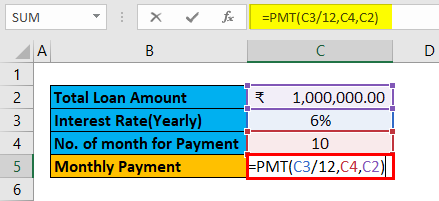The result is given below: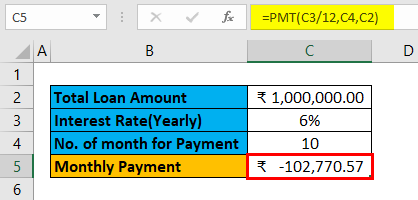Step 1: Write down the desired months input in column E and Loan amount in row 4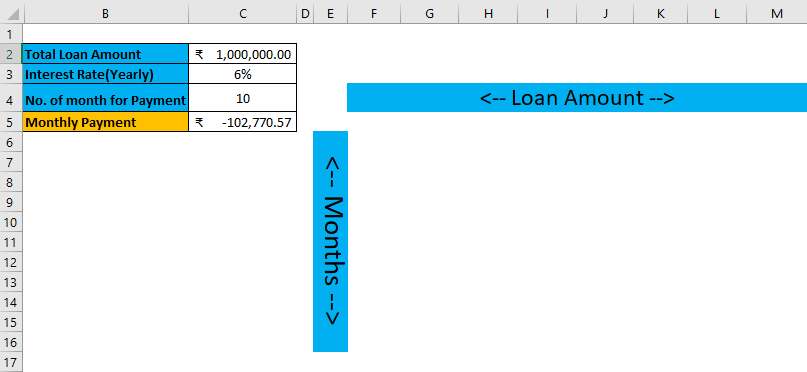Step 2: Enter all user desired months in column F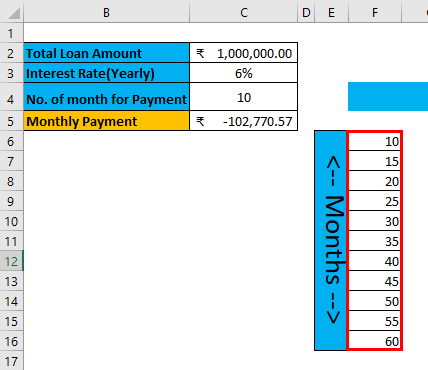Step 3: Enter all desired loan amount in the 5th row of a sheet.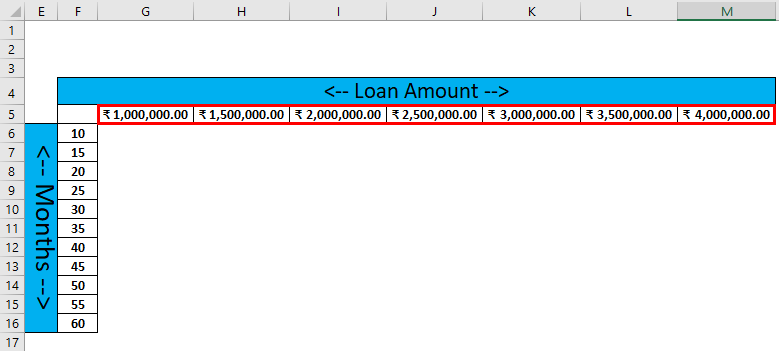Step 4: Select cell F5 and the assigned value of the cell C5, click Enter.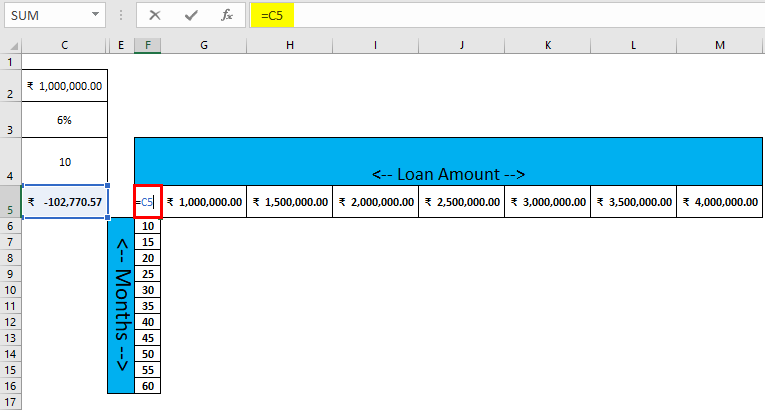Step 5: Now select all dataset from F5 to F16 and F5 to M5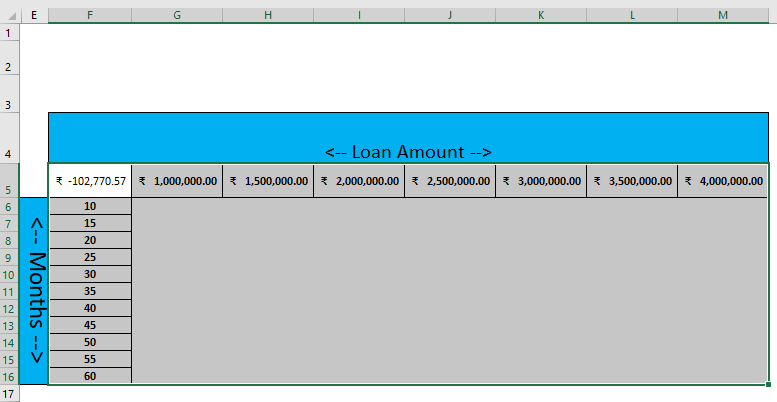Step 6: Go to Data tab > click on the What-if Analysis drop-down list toolbar > select Data Table.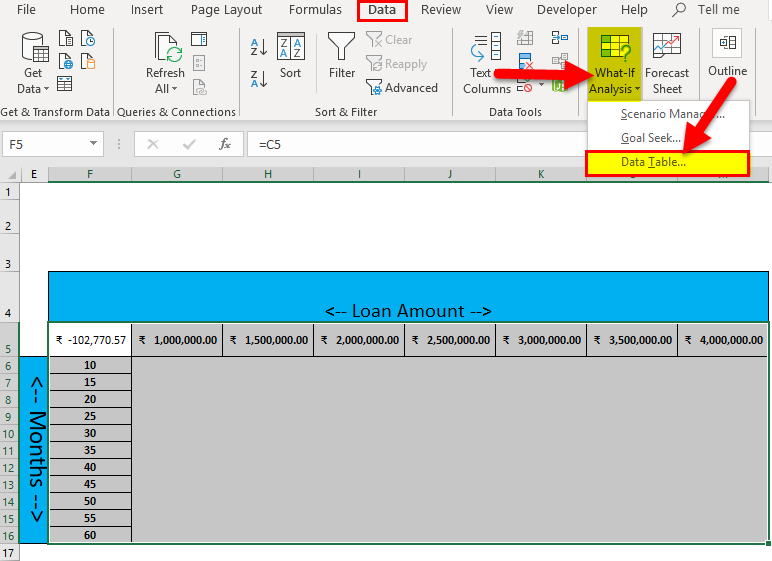Step 7: Data Table pop up will appear with two input cells for row and column >Select Loan amount in the Row input cell and no. of months for Payment in Column input cell > click OK button.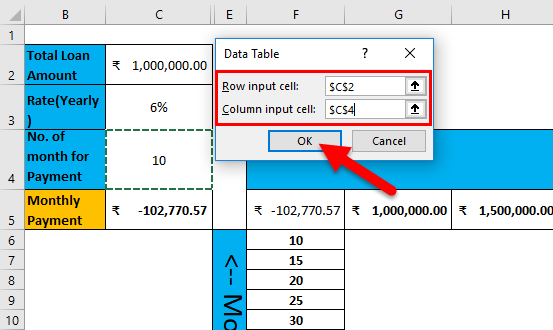Step 8: Result will be published per month loan re-payment amount as per combination of each month and loan amount data set.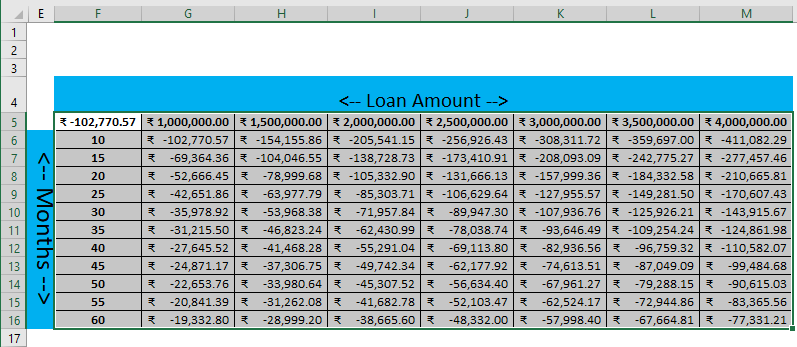Summary of Example #1

As we can see the data table for each month and Loan amount. If a user wants to take ₹   2,000,000.00 loans then he needs to pay  ₹ -105,332.90 for next 20 Months as EMI.

The same amount if a person wants to pay for the next 30 months then  ₹ -38,665.60 Need to pay as EMI. There are so many scenarios for multiple months and respective re-pay the amount as EMI.

### Two-Variable Data Table in Excel Example #2

Create a Two-Variable data table for the different Loan Amount and Interest rate

Pre-requisite: Write down a sample calculation. As mentioned in the workbook Example #2. Assume a Total loan amount i.e.₹ 1,000,000.00, Interest Rate (Yearly) i.e. 2%, Number of the month for EMI / Payment i.e. 1o Month and apply PMT formulas (=PMT (C3/12, C4, C2)) to calculate the EMI.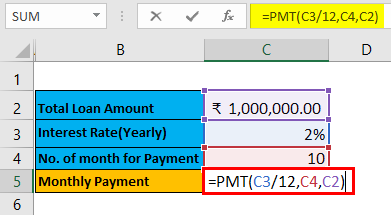The result is given below: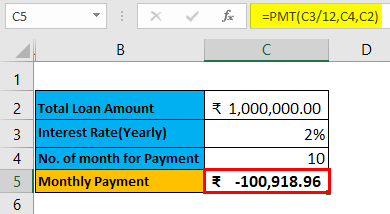Step 1: Write down desired Interest rate input in the column D and Loan amount in row 3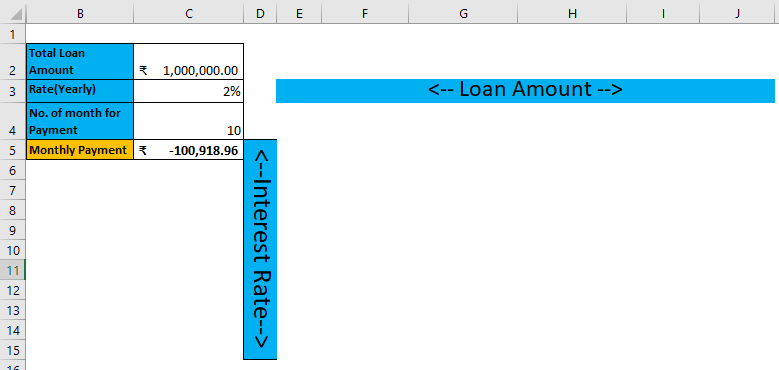Step 2: Enter all user desired interest rates in the E column.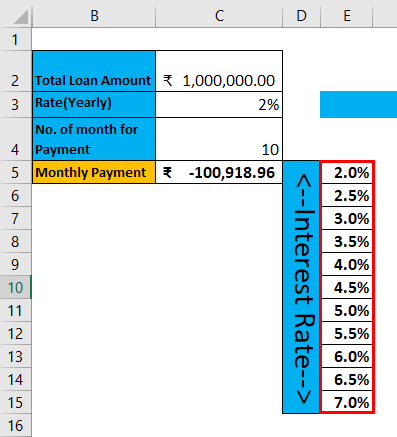Step 3: Enter all desired loan amount in the 4th row of the sheet.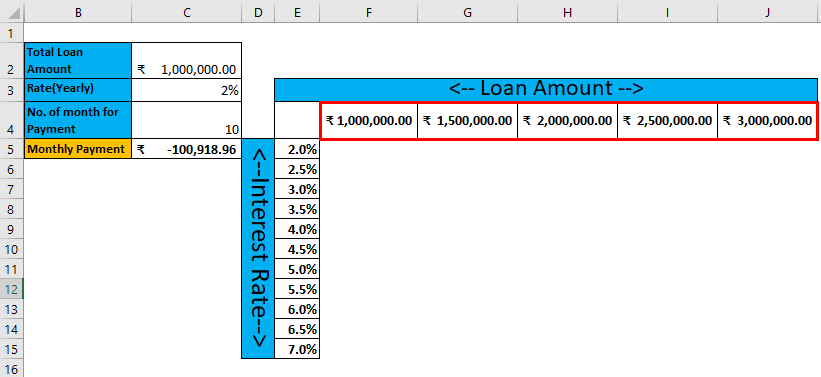Step 4: Select cell E4 and the assigned value of cell C5, click Enter.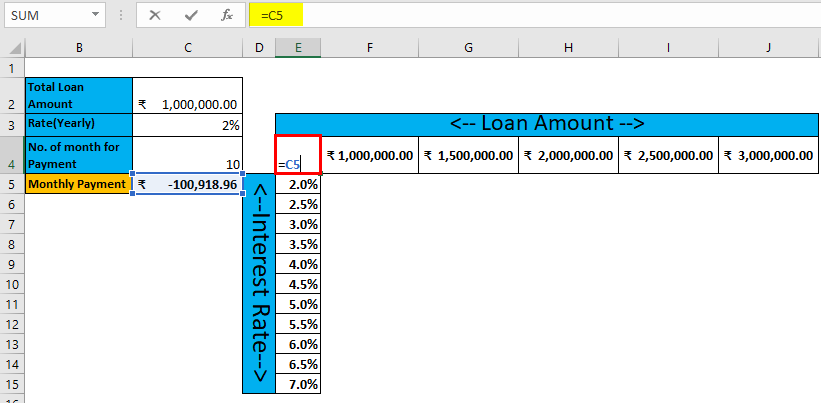Step 5: Now select all dataset from E4 to E15 and E4 to J4.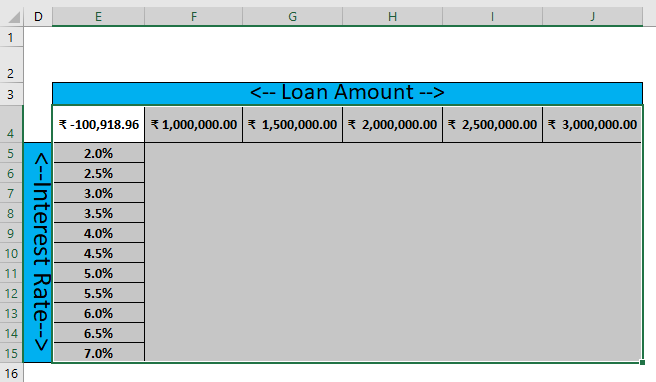Step 6: Go to Data tab > click on the What-if Analysis drop-down list toolbar > select Data Table.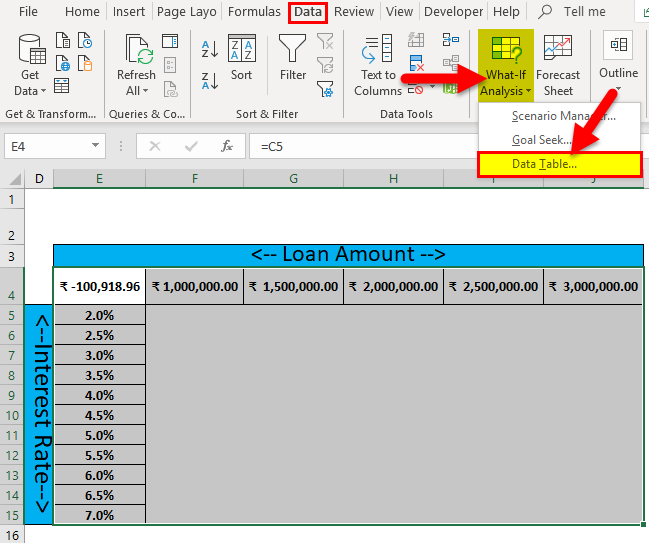Step 7: Data Table pop up will appear with two input cells for row and column >Select Loan amount in the Row input cell and interest rate in Column input cell > click ok button.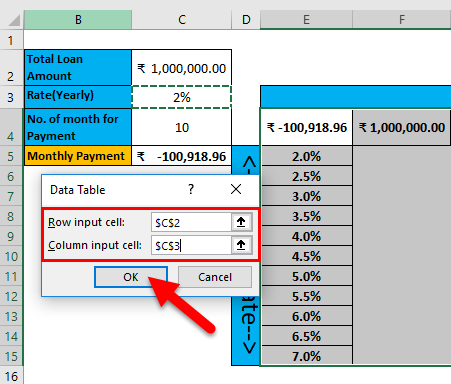Step 8: Result will be published per month loan re-payment amount as per combination of different Interest rate and loan amount data set.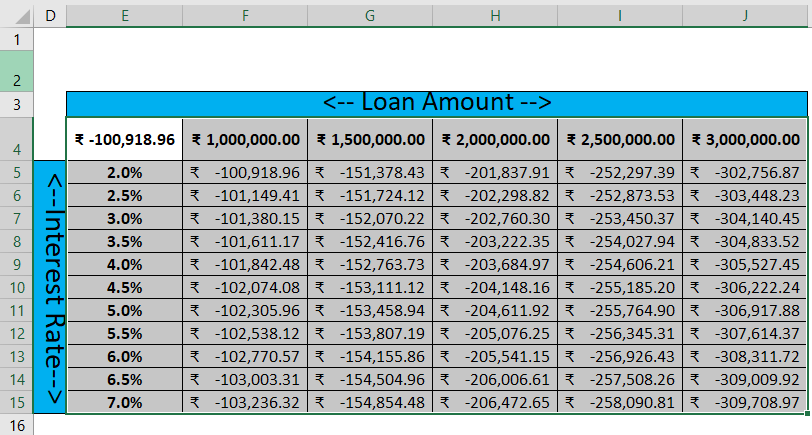Summary of Example #2

As we can see the data table for each Interest rate and Loan amount as result. Like if a user wants to take ₹   2,000,000.00 loans then he needs to pay as EMI  ₹  -203,684.97 for 4% of interest rate to the next 10 months.

The same amount if a person wants to pay for the next 3% interest rate then  ₹ -202,760.30 Need to pay as EMI. There are so many scenarios for multiple Interest rate and respective re-pay amount as EMI.

### Component of Data Table

1. Row Input Cell: Enter the value for which a user filled in a row and want to see analyzed result.
2. Column Input Cell: Enter the value for which a user filled in a Column and want to see analyzed result.

When to use Two Variables Data Table:

• If there are multiple scenarios for two input variables, then prefer two-variable data table tools.
• If a user wants to use PMT function, then Data table will be best for it to see multiple results on changing input values.
• For loan calculation, most people want to see EMI after changing the month and the Loan amount for the constant interest rate.
• If a user wants to change the value of the constant variable, it will reflect for all the results array of the data table.

### Limitation of the Data Table in Excel

1. It cannot use more than two variables as an input parameter for analysis, because it has the limitation of either use one variable or two variables.
2. Data tables are calculated each time when a user opens a workbook which has a data table. It will not matter that user doing any changes in the workbook or not, to save this a user need to change the calculation option to Automatically Recalculate the workbook, not the data table.
3. After applying the data table, the resultant table a user cannot edit or delete a single result because the result is an array. It will throw an error “Cannot change part of a data table.”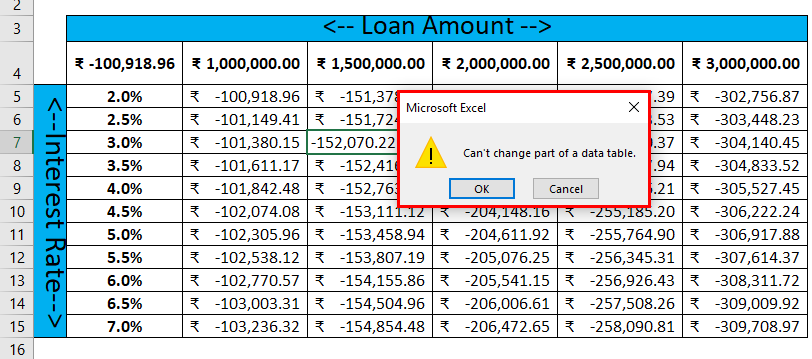### Things to Remember About Two Variables Data Table in Excel

If a user wants to edit or delete a part of the result from the data table, then he needs to select the entire array range and then press Delete key on the keyword. If the user wants to do undo or Ctl+Z it will not work, and user needs to go with the previous method.

You can download this Two-Variable Data Table Excel template here –Two Variable Data Table Excel Template

### Recommended Articles

This has been a guide to Two Variable Data Table in Excel. Here we discuss how to create Two Variable data table examples and downloadable excel templates. You may also look at these useful functions in excel –

1. Guide to ISERROR Excel Function
2. How to Use MIRR Excel Function?
3. RANK Excel Function – You Must Know
4. Excel T.Test Function in MS Excel

The post Two Variable Data Table in Excel appeared first on EDUCBA.

This post first appeared on Best Online Training & Video Courses | EduCBA, please read the originial post: here